﻿ 分块矩阵的应用研究

# 分块矩阵的应用研究Research on the Application of Block Matrix

Abstract: In advanced mathematics, block matrix is a very important concept. It can make the representation of matrix more simple and clear, and simplify the operation of matrix. In this paper, through the simple analysis of the calculation and proof of block matrix, we discuss the method of matrix with n-order special attribute, and use block matrix to describe the solution of linear system and its related content.

1. 引言

${a}_{1}x+{b}_{1}y+{c}_{1}z={d}_{1},$ (1.1)

${a}_{2}x+{b}_{2}y+{c}_{2}z={d}_{2},$ (1.2)

${a}_{3}x+{b}_{3}y+{c}_{3}z={d}_{3},$ (1.3)(1.4)

2. 分块矩阵在计算方面的应用

2.1. 逆矩阵方面的应用

$P=\left[\begin{array}{cc}A& B\\ C& D\end{array}\right]$

B是r阶，C是k阶，当B与( $C-D{B}^{-1}A$ )两个方阵是可逆的，那么P也是可逆的，

1) 当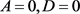，B与C都可逆时，有 ${P}^{-1}=\left[\begin{array}{cc}0& {C}^{-1}\\ {B}^{-1}& 0\end{array}\right]$

2) 当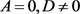，B与C都可逆时，有 ${P}^{-1}=\left[\begin{array}{cc}-{C}^{-1}D{B}^{-1}& {C}^{-1}\\ {B}^{-1}& 0\end{array}\right]$

3) 当 $A\ne 0,D\ne 0$，B与C都可逆时，有 ${P}^{-1}=\left[\begin{array}{cc}0& {C}^{-1}\\ {B}^{-1}& -{B}^{-1}A{C}^{-1}\end{array}\right]$

$Q=\left[\begin{array}{cc}A& B\\ C& D\end{array}\right]$

${Q}^{-1}={\left[\begin{array}{cc}A& B\\ C& D\end{array}\right]}^{-1}=\left[\begin{array}{cc}{A}^{-1}+{A}^{-1}B{\left(D-C{A}^{-1}B\right)}^{-1}C{A}^{-1}& -{A}^{-1}B{\left(D-C{A}^{-1}B\right)}^{-1}\\ -{\left(D-C{A}^{-1}B\right)}^{-1}C{A}^{-1}& {\left(D-CAB\right)}^{-1}\end{array}\right]$

1) 当 $B=0,C=0$，A与D都可逆时，有 ${Q}^{-1}=\left[\begin{array}{cc}{A}^{-1}& 0\\ 0& {D}^{-1}\end{array}\right]$

2) 当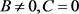，A与D都可逆时，有 ${Q}^{-1}=\left[\begin{array}{cc}{A}^{-1}& -{A}^{-1}B{D}^{-1}\\ 0& {D}^{-1}\end{array}\right]$

3) 当 $B=0,C\ne 0$，A与D都可逆时，有 ${Q}^{-1}=\left[\begin{array}{cc}{A}^{-1}& 0\\ -{D}^{-1}C{A}^{-1}& {D}^{-1}\end{array}\right]$

$M=\left(\begin{array}{cccccc}0& {m}_{1}& 0& \cdots & 0& 0\\ 0& 0& {m}_{2}& \cdots & 0& 0\\ ⋮& ⋮& ⋮& & ⋮& ⋮\\ 0& 0& 0& \cdots & 0& {m}_{n-1}\\ {m}_{n}& 0& 0& \cdots & 0& 0\end{array}\right)\left({m}_{i}\ne 0,i=1,\cdots ,n\right)$

$N=\left(\begin{array}{ccccc}{m}_{1}& 0& \cdots & 0& 0\\ 0& {m}_{2}& \cdots & 0& 0\\ ⋮& ⋮& & ⋮& ⋮\\ 0& 0& \cdots & 0& {m}_{n-1}\end{array}\right)$

${M}^{-1}=\left(\begin{array}{cc}0& {m}_{n}^{-1}\\ {N}^{-1}& 0\end{array}\right),{N}^{-1}=\left(\begin{array}{ccccc}{m}_{1}^{-1}& 0& \cdots & 0& 0\\ 0& {m}_{2}^{-1}& \cdots & 0& 0\\ ⋮& ⋮& & ⋮& ⋮\\ 0& 0& \cdots & 0& {m}_{n-1}^{-1}\end{array}\right)$

${M}^{-1}=\left(\begin{array}{cccccc}0& 0& 0& \cdots & 0& 1/{m}_{n}\\ 1/{m}_{1}& 0& 0& \cdots & 0& 0\\ ⋮& ⋮& ⋮& & ⋮& ⋮\\ 0& 0& 0& \cdots & 0& 0\\ 0& 0& 0& \cdots & 1/{m}_{n-1}& 0\end{array}\right)$

2.2. 行列式计算方面的应用

$H=\left[\begin{array}{cccc}{P}_{1}& & \cdots & P\\ 0& {P}_{2}& & ⋮\\ ⋮& ⋮& \ddots & \\ 0& 0& \cdots & {P}_{s}\end{array}\right]$

$|P|=\left[\begin{array}{ccccccc}x& 0& 0& \cdots & 0& 0& z\\ 0& x& 0& \cdots & 0& z& 0\\ 0& 0& x& \cdots & z& 0& 0\\ ⋮& ⋮& ⋮& & ⋮& ⋮& ⋮\\ 0& 0& z& \cdots & x& 0& 0\\ 0& z& 0& \cdots & 0& x& 0\\ z& 0& 0& \cdots & 0& 0& 0\end{array}\right]$

$A=\left[\begin{array}{cccc}x& & & \\ & x& & \\ & & \ddots & \\ & & & x\end{array}\right]=D,\text{\hspace{0.17em}}\text{\hspace{0.17em}}B=\left[\begin{array}{cccc}& & & z\\ & & z& \\ & ⋰& & \\ z& & & \end{array}\right]=C$

$|P|=|\begin{array}{cc}A& B\\ C& D\end{array}|=|A|\cdot |D-C{A}^{-1}B|$$|P|={x}^{n}{\left(x-{z}^{2}{x}^{-1}\right)}^{n}={\left({x}^{2}-{z}^{2}\right)}^{n}$

2.3. 矩阵特征值方面的应用

$\left[\begin{array}{cc}{E}_{r}& 0\\ 0& 0\end{array}\right]$

$\left[\begin{array}{cc}0& 0\\ 0& {E}_{r}\end{array}\right]$

1) 当 $R\left(A\right)=0$ 时，即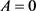，结论显然成立。

2) 设 $0，非零不可逆矩阵A，因为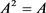，所以有可逆矩阵P，

3. 分块矩阵在证明方面的应用

$R\left({A}_{s}\right)\ge R\left(A\right)+s-m$

$R\left(A+B\right)\le R\left(A\right)+R\left( B \right)$

$R\left[\begin{array}{c}0\\ B\end{array}\right]=R\left({A}_{s}\right)+m-s$

$T=P\left[\begin{array}{cc}{E}_{r}& 0\\ 0& 0\end{array}\right]Q=P\left[\begin{array}{c}{E}_{r}\\ 0\end{array}\right]\left(\begin{array}{cc}{E}_{r}& 0\end{array}\right)Q={T}_{1}{T}_{2}$

${T}_{1}=P\left[\begin{array}{c}{E}_{r}\\ 0\end{array}\right],\text{\hspace{0.17em}}{T}_{2}=\left(\begin{array}{cc}{E}_{r}& 0\end{array}\right)Q$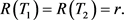居余马. 线性代数[M]. 北京: 清华大学出版社, 2005.

 北京大学数学系几何与代数教研室前代数小组编. 高等代数[M]. 第三版. 北京: 高等教育出版社, 2007.

 王祖朝, 褚宝增. 线性代数[M]. 北京: 北京师范大学出版社, 2008.

 黄承绪, 徐德余. Jordan标准形与矩阵最小多项式[J]. 绵阳师范高等专科学校学报, 1994(S1): 27-29.

 王超亚. 分块矩阵的若干初等运算及应用[J]. 计算机光盘软件与应用, 2013(5): 161-162.

 王莲花, 李念伟, 梁志新. 分块矩阵在行列式计算中的应用[J]. 河南教育学院学报(自然科学卷), 2005, 14(3): 12-15.

 曾聃, 徐运阁. 矩阵的逆及秩的降阶方法[J]. 大学数学, 2019, 35(5): 117-121.

 曹重光. 体上分块矩阵群逆的某些结果[J]. 黑龙江大学自然科学学报, 2001(3): 5-7.

 Ma, C. (2019) Normal Completions of Block Partial Matrices. Indian Journal of Pure and Applied Mathematics, 50, 353-363.
https://doi.org/10.1007/s13226-019-0330-y

 Gumus, M., Liu, J.Z., Raouafi, S. and Tam, T.-Y. (2018) Positive Semi-Definite 2 × 2 Block Matrices and Norm Inequalities. Linear Algebra and Its Applications, 551, 83-91.
https://doi.org/10.1016/j.laa.2018.03.046

 Mosić, D. and Djordjević, D.S. (2018) Block Representations of the Generalized Drazin Inverse. Applied Mathematics and Computation, 331, 200-209.
https://doi.org/10.1016/j.amc.2018.03.027

Top